# Research

Systems of interacting agents arise in a wide variety of disciplines, including Physics, Biology, Ecology, Neurobiology, Social Sciences, Economics, … Agents may represent elementary particles, atoms, cells, animals, neurons, people, rational agents, opinions, etc…
The understanding of agent interactions at the appropriate scale in these systems is as fundamental a problem as the understanding of interaction laws of particles in Physics.
We propose a non-parametric approach for learning interaction laws in particle- and agent-based systems, based on observations of trajectories of the states (e.g. position, opinion, etc…) of the systems, with the crucial assumption (for now) that the interaction kernel depends on pairwise distances only. Unlike recent efforts, we do not require libraries of features or parametric forms for such interactions.

Relevant publications

Code

Some videos of interacting particle/agent systems. If they do not start automatically, please right click and start the videos. Insets: Cucker-Smale flocking (left), fish mills (right).

In each inset: Left: trajectories of true system, from an initial condition used during the training phase (top) and a new random initial condition (bottom). Right: trajectories of the system with the learned interaction kernel, started from the same initial conditions as the corresponding system on the left.

More examples, movies, etc…available on M. Zhong’s webpage

CONFERENCES/WORKSHOPS

Second Symposium on Machine Learning and Dynamical Systems, Fields Institute, Toronto, Sep. 21-25, 2020
Understanding Many-Particle Systems with Machine Learning, IPAM, Fall 2016
Symposium on Machine Learning and Dynamical Systems, Imperial College London, Feb. 11-13, 2019
Validation and Guarantees in Learning Physical Models: from Patterns to Governing Equations to Laws of Nature, IPAM, Fall 2019, part of the program Machine Learning for Physics and the Physics of Learning

We have developed several methods for analyzing in a multiscale fashion the geometry of data in high-dimensions, when the data is close to being low-dimensional. In particular we developed a technique we called Multiscale SVD, where the main idea is to fix a point and look at the behavior of singular values of the covariance of the data in a ball of radius r centered at the point, as r varies. This behavior is useful to reveal the local intrinsic dimension of the data, is robust to noise and sampling, and also can be used to estimate the largest region around the point which is approximately low-dimensional. This is useful in a wide variety of applications. We have used it to analyze molecular dynamics data, and construct reduced models, to estimate the intrinsic dimension of a variety of data sets, and estimate K-flats approximations to data efficiently. We have also developed a technique called Geometric Multi-Resolution Analysis, a.k.a. GMRA, which we use to construct piecewise linear multiscale approximations to data. This leads to a novel way of approximating data, of decomposing data matrices in a hierarchical fashion, of constructing dictionaries that sparsify data, and we use it as a scaffold to efficiently encode data and on which to run a variety of estimation tasks (from regression to classification to model reduction for stochastic systems) efficiently (both from a sample complexity perspective and a computational perspective). Papers

Diffusion wavelets generalize classical wavelets, allowing for multiscale analysis on general structures, such as manifolds, graphs and point clouds in Euclidean space. They allow to perform signal processing tasks on functions on these spaces. This has several applications. The ones we are currently focusing on arise in the study of data sets which can be modeled as graphs, and one is interested in learning functions on such graphs. For example we can consider a graph whose vertices are proteins, the edges connect interacting proteins, and the function on the graph labels a functionality of the protein. Or each vertex could be an image (e.g. a handwirtten digit), the edge connect very similar images, and the function at each vertex is the value of digit represented by that vertex.

In classical, one-dimensional wavelet theory one applies dilations by powers of 2 and translations by integers to a mother wavelet, and obtains orthonormal wavelet bases. The classical construction has been of course generalized in many ways, considering wide groups of transformations, spaces different from the real line, such as higher-dimensional Euclideanspaces, Lie groups, etc…Most of these constructions are based on groups of geometrical transformations of the space, that are then applied (as a “change of variable”) to functions on that space to obtain wavelets.

On general graphs, point clouds and manifolds there may not be nice or rich groups of transformations. So instead of assuming the existence of these “symmetries” we directly use semigroups of operators acting on functions on the space (and not on the space itself). We typically use “diffusion operators”, because of their nice properties. Let T be a diffusion operator on a graph T (e.g. the heat operator). In many cases, our graph will be a discretization of a manifold. The study of the eigenfunctions and eigenvalues of T is known as Spectral Graph Theory and can be viewed (for our purposes) a generalization of the classical theory of Fourier series on the circle. The advantages of wavelets and multi-scale techniques over classical Fourier series are well known. So it is natural to attempt to generalize the wavelet theory to the setting of diffusion operators on graphs. Following Stein, we take the view that the dyadic powers of the operator T establish a scale for performing multiresolution analysis on the graph. In the paper, “Diffusion Wavelets,” we introduce a procedure for construction scaling functions and wavelets adapted to these scales.

## Sketch of the Construction

In several applications there is a need to organize structures in a multi-resolution fashion, in order to process them, understand them, compress them etc…Maybe the most classical example is given by Fourier analysis, where different resolutions correspond to different frequency bands in which a signal can be analyzed. However, wavelets allow one to perform a multi-resolution analysis in a somehow stronger and better organized way in the spatial domain. The construction of wavelets in Euclidean spaces is by now in many ways quite well understood, even if interesting open questions remain for higher-dimensional wavelet constructions. Wavelets have found also wide applications in numerical analysis, both as mathematical foundation of Fast Multipole Methods, and by providing bases for Galerkin methods. Already in this latter setting, though, higher flexibility has been required than low-dimensional Euclidean wavelets, since multi-resolution are needed on rather general domains and manifolds. Both the Fast Multipole Methods and the Galerkin wavelet methods are not very well adapted to the operator, and only through some efforts are they adapted to the geometry of the domain/manifold. Finally, we want to mention the setting of Spectral Graph Theory, where one can naturally do Fourier analysis through the Laplacian of a graph, and several multi-scale constructions, for use in a variety of applications, are still quite ad hoc.
In the paper “Diffusion Wavelets” we propose a construction of wavelets on discrete (or discretized continuous) graphs and spaces, that are adapted to the “geometry” of a given diffusion operator T, where the attribute “diffusion” is intended in a rather general sense. The motivation for starting with a given diffusion operator is that in many cases one is interested in studying functions on the graph/space, and hence it seems natural to start with a local operator generating local relationships between functions. Powers of the operator propagate these relationships further away till they become global.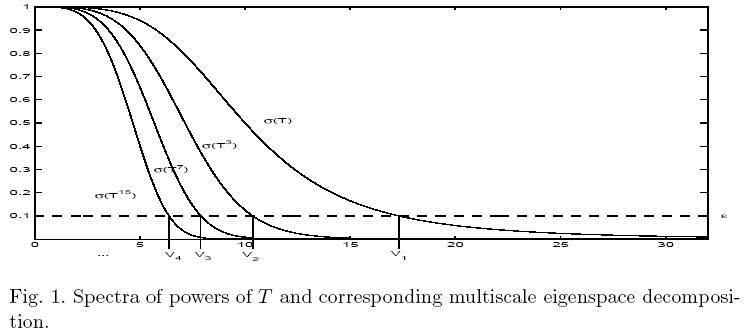If the spectrum of T decays, large powers have low-rank, and hence are compressible. For example we can think of the range of high power of T as being essentially spanned by very smooth functions with small gradient, or even band-limited functions. It is natural to take advantage of this, and compress the ranks of (dyadic) powers of the operator, thus obtaining a decreasing chain of subspaces, which can be interpreted as scaling function approximation spaces. The difference between these subspace can be called wavelet subspaces. The construction of the basis elements is non-trivial: we show one can build orthonormal bases of scaling functions and wavelets, with good localization properties in about order n(log n)^2, even if the constants are still big and their improvement is of great practical significance and is being investigated.

The algorithm proceeds by applying T^(2^j), expressed on the basis of orthonormal scaling functions spanning V_j, then orthogonalizing the resulting set of vectors, discarding the ones not needed to span (numerically) the same subspace, and so on. Hence the orthonormalization step encapsulate the downsampling step.
Our construction works on a Riemannian manifold, with respect to, for example, the Laplace-Beltrami diffusion on the manifold; on a weighted graph, with respect, for example, to the natural diffusion induced by the weights on graph.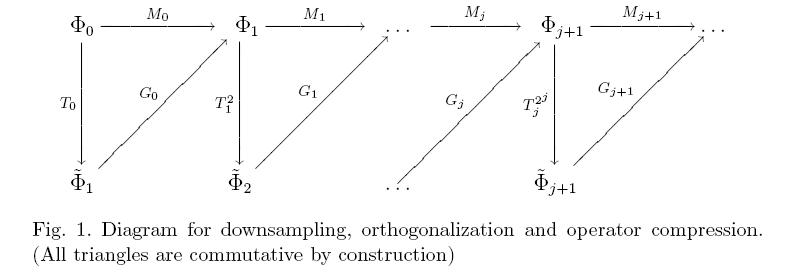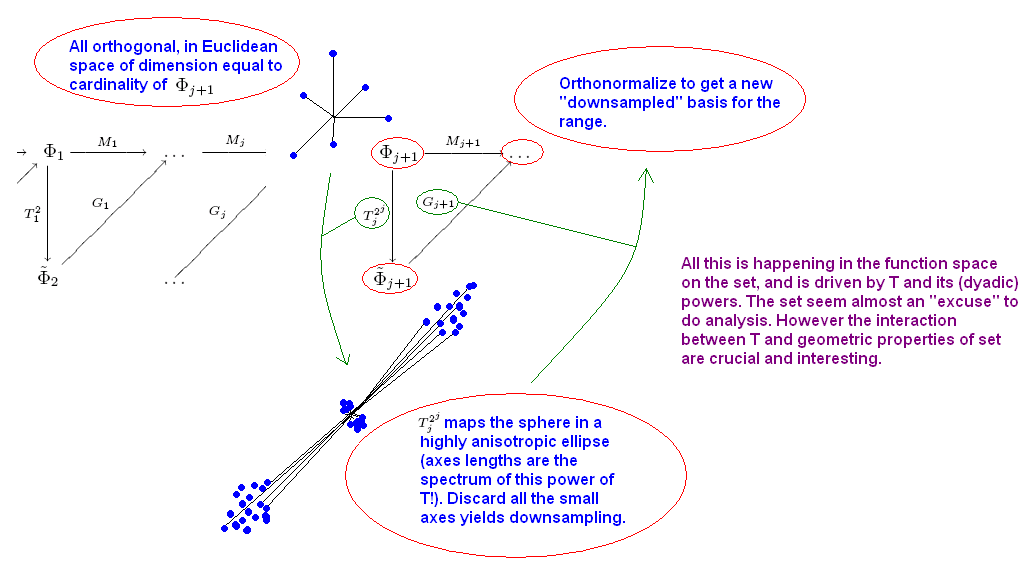### Examples

#### Homogeneous diffusion on a circle

The first example is a standard diffusion on the circle: our set is the circle sampled at 256 points, an initial orthonormal basis is given by the set of 256 delta-functions at each points, and the diffusion is given by the standard homogeneous diffusion on the circle. In the picture, we plot several diffusion scaling functions in various scaling function spaces. The finest scaling functions are the given delta-functions. These diffuse in ‘triangle functions’ (linear splines): these are orthonormalized into a (non-translation invariant) basis of ‘triangle functions’ and linear combinations of ‘triangle functions’. Those are diffuse again twice etc.. The coarse scaling function spaces are spanned by the first few top eigenfunctions of the diffusion operator, which are simply trigonometric polynomials with small frequency. The algorithm still tries to build well-localized scaling functions out these trigonometric polynomials (see e.g. V_9, V_10, V_11) when possible.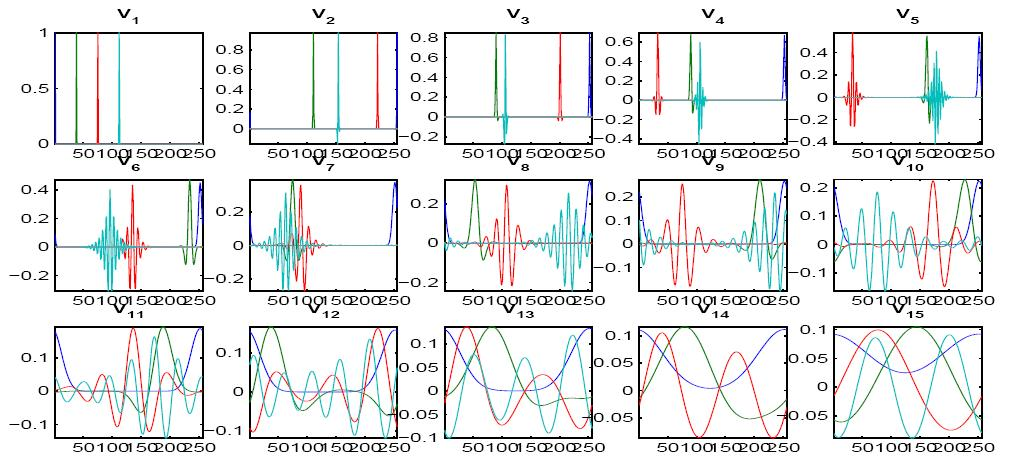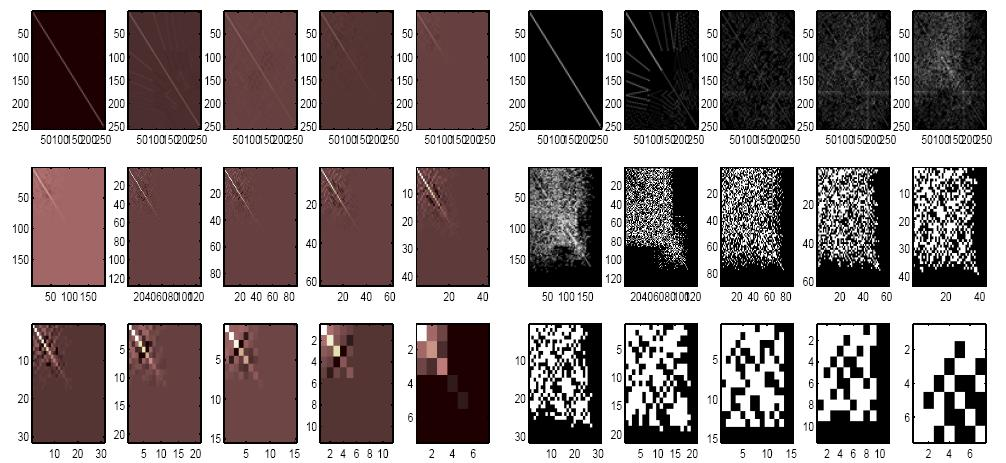On the left we plot the compressed matrices representing powers of the diffusion operator, on the right we plot the entries of the same matrices which are above precision. The initial powers get fuller because the spectrum of the diffusion is slowly decaying. It is enough to consider, instead of the given diffusion, a small power of it to avoid this partial fill-up.

#### Non-homogeneous diffusion on the circle

We consider a circle as before, but now the diffusion operator is not translation invariant or homogeneous: the conductivity is non-constant and is depicted in the figure in the top-left position. Think of the circle of different materials, which is most conductive at the top and least conductive (almost insulating material) at the bottom. In the top right picture we represent some scaling functions at level 15, so by construction/definition, these scaling functions span the range of T^(2^16-1). Observe that the “scale” of these scaling functions is far from uniform if we measure it in a translation invariant way (for example with standard wavelets on the circle, or with the diffusion wavelets of the previous example). However this is exactly the scale at which the corresponding power of T is operating: at the top of the circle the diffusion acted fast , the numerical rank of the operator restricted to that region is very small, and the scaling functions are trivial there; on the bottom part of the circle, this power of T is still far from trivial, since there the diffusion is much slower, and scaling functions exhibit a more complex behavior (they still contain local high-frequency components).
In the second row we show how one can compute an eigenfunction of the compressed operator and extend it back to the whole space, with good precision.
In the third row we show the entries above precision of a power of the operator (left); on the right we show a “diffusion scaling function” embedding, which shows that points at the bottom of the circle have a large “diffusion distance” (it is hard/takes a long time to diffuse from one to the other) while points at the top have a small diffusion distance. Diffusion distance in original space is roughly Euclidean distance in this “diffusion scaling function” embedding.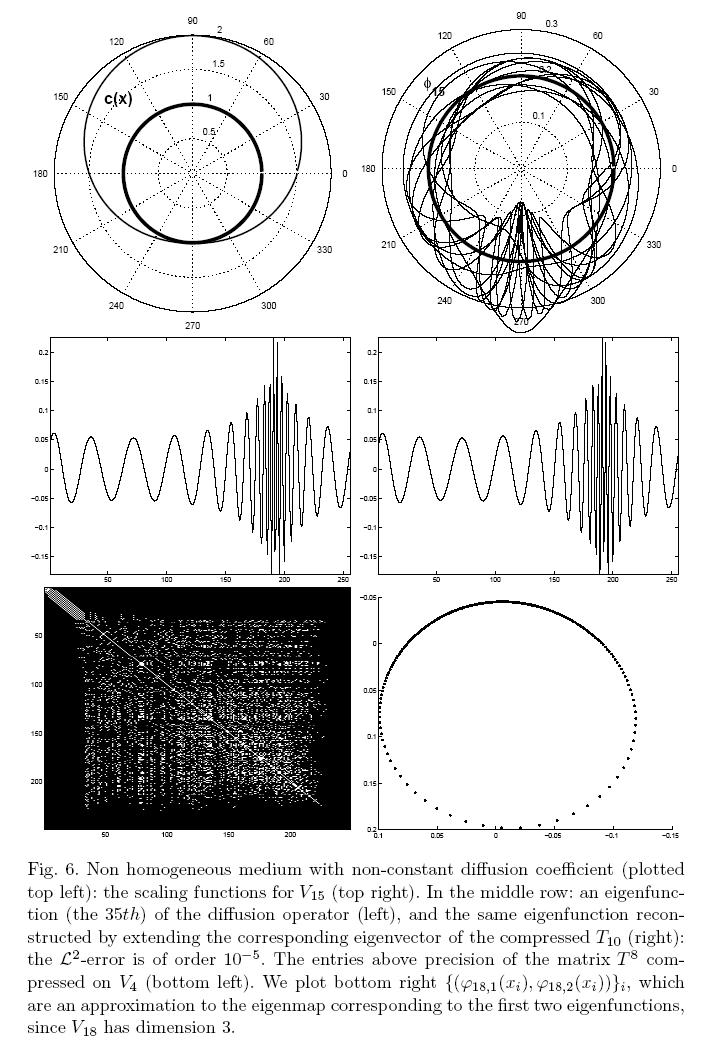#### Diffusion on a graph of 3 Gaussian clouds

In this example we consider the graph induced by points randomly distributed according to three Guassian random variables centered at different points. A graph is associated to these points, by putting edges between close-by points, with weights which are an exponentially decays function of the distances between the points.
The picture top-left shows the points, the picture top center shows the image of the points under the Laplacian eigenfunction map, the remaining figures show some diffusion scaling functions and wavelets, hinting at how they could be used to localized the analysis of this graph.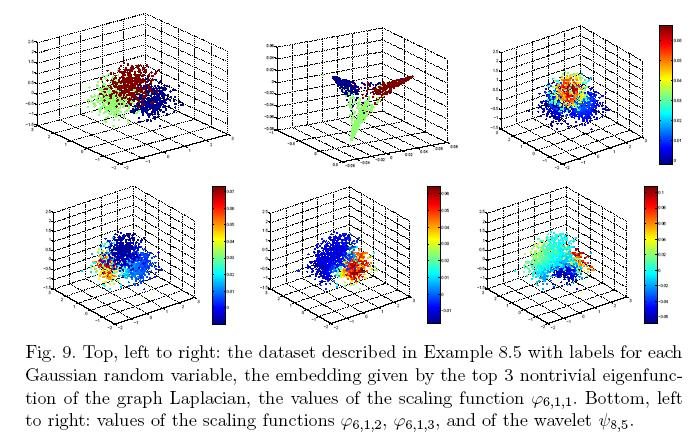#### Diffusion a dumbbell-shaped manifold

We consider a dumbbell-shaped manifold, with diffusion given by (a discrete approximation to) the Laplace-Beltrami operator.
The picture shows different scaling functions and wavelets at different scales, on this manifold.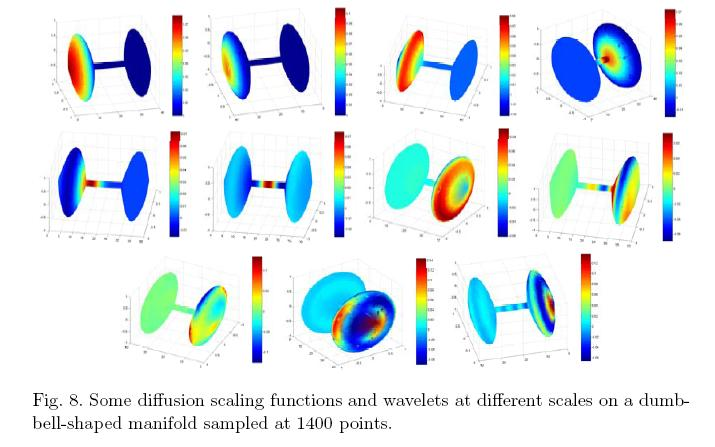#### Extension outside the set

We also propose an algorithm for extending scaling functions and wavelets from the data set. In the figure we show the extension of some scaling functions from the set (left) to many points randomly generated around the set.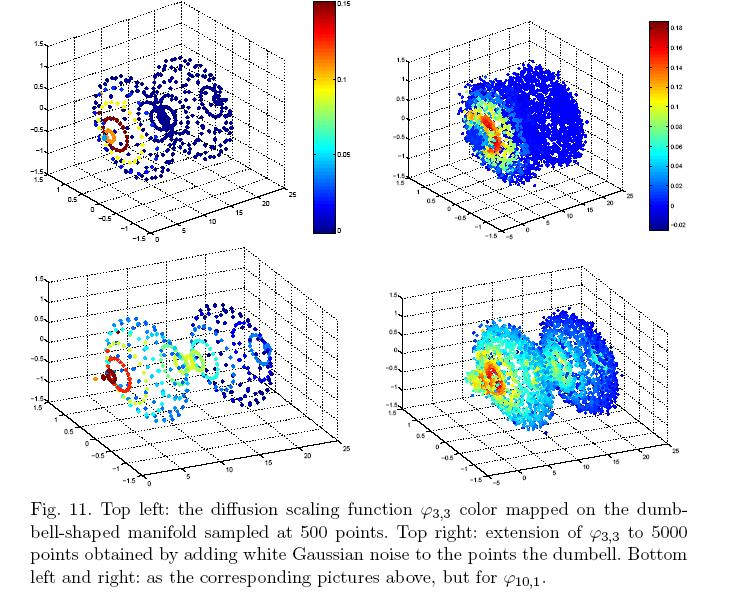## Diffusion Wavelet Packets

Diffusion Wavelet Packets can be constructed by further splitting the wavelet subspace further, as in the classical case.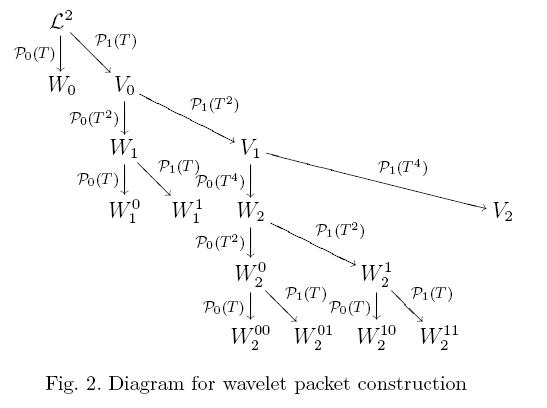## Local Discriminant Bases with Diffusion Wavelet Packets

We consider two classes of functions on the sphere, and the problem of learning a good set of features such that the projection on them allows for good discrimination between the functions of the two classes.
Functions of class A are build as the superposition of three ripply functions, with equi-oriented ripples of the same frequency, centered around three points moving slightly in a noisy way, and two Guassian bumps acting as decoys, which are non-overlapping with the three ripply functions. Functions of class B are similar but one (randomly chosen) ripply function has ripples in a different direction than the other two.
Running CART on the original data has an error of 48%, running it on the top 40 LDB features leads to an error of about 12.5%, and running it onto the top discriminating eigenfunctions leads to an error of about 18%.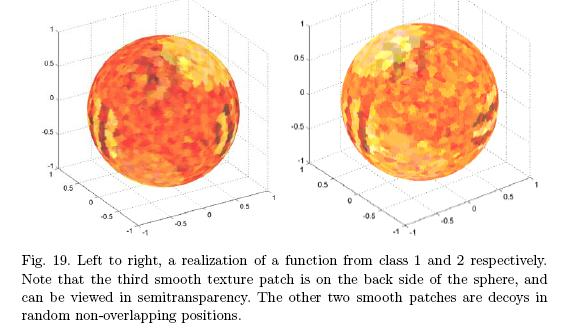As a second example of local discriminant basis, we consider the following two classes of functions. We fix a direction v, and slightly perturb it with random Gaussian noise. Around that direction we create a ripply spherical cap, with one or two oscillation depending on the class. We add then 5 non-overlapping ripply functions, acting as decoy, randomly on the sphere.
CART run on the original data has an error of about 17.5%, CART on the top 20 LDB features has an error of about 3.5%, and CART on the first 300 eigenfunctions has an error of about 31%.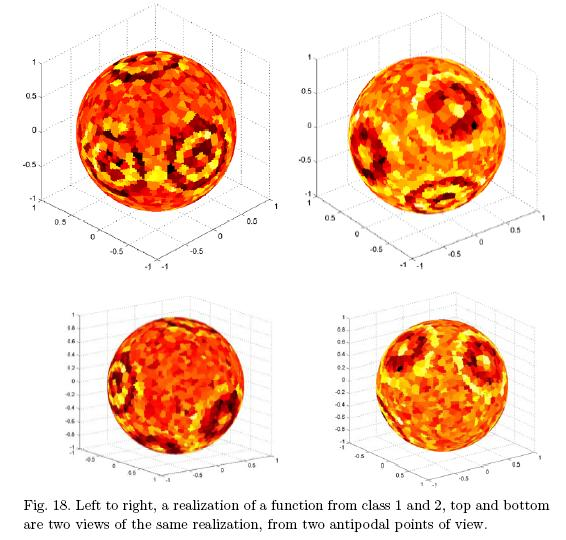Diffusion geometry refers to the large-scale geometry of a manifold or a graph representing a data set, which is determined by long-time heat flows on the manifold/graph/data set.

A multiscale analysis, that includes all scales and not just large scales, is possible with diffusion wavelets.

This behavior can be accurately described by the bottom eigenfunctions of the Laplacian operator on the manifold or on the graph (or data set). Moreover, these eigenfunctions can be used to define an embedding (sometimes called eigenmap) of the manifold or graph into low-dimensional Euclidean space, in such a way that Euclidean distances in the range of the eigenmap correspond to “diffusion distances”; on the manifold or graph. Techniques based on eigenvectors of similarity matrices (which are related to the Laplacian have been used successfully for some time in several problems in data analysis, segmentation, clustering, etc…
These connections, and connection to differential-geometric structures of manifolds, and
problems related to the stability, computability, and extendibility of these eigenfunctions has been explored extensively in Stephane Lafon’s thesis.
Further material, including talks and papers, are available on Stephane Lafon’s web-page.

Diffusion Geometry was introduced with R. Coifman and Stephane Lafon (now at Google – check his home page for cool demos introducing the basics of diffusion geometries!).
There a few key ideas behind the introduction of Diffusion Geometry.

• The first key idea is that for high-dimensional data large distances are often not reliable, as they are severaly corrupted by noise, and if data has low-dimensional geometry, large distances do not respect such geometry.
Therefore one starts by connecting each data point only with the closest points – those close enough for which the distance may be trusted. This leads to constructing a graph where each point is connected to its nearest point, forming a graph, possibly weighted in such a way that closest points are connected by an edge with ggreater weight.
• The second key idea is that even if the data lies on a low-dimensional manifold, the geodesic (i.e. shortest path) distance on the manifold is not necessarily an effective distance, as it may be too easily be corrupted by noise.
In diffusion geometry the geodesic distance is replaced by diffusion distance, which is in fact a family of distances, paramtrized by time. Diffusion distance between two points at time t takes into considerations all paths of length up to t in order evaluate a distance between the two points, weighting each path by the probability of realizing that path according to the random walk on the graph of nearest points previously constructed.
• The third idea is that one may create a map from high-dimensional space to low-dimensional sapce, that respects diffusion distance, by using the top eigenvectors of the random walk on the graph. This is essentially kernel PCA, with the kernel being the random walk on the graph – in particular the kernel is data-adaptive.
• The fourth idea is that this constrution may be multiscaled. With diffusion wavelets, this idea is carried further, to consider multiple scales on the graph in a multi-resolution fashion.

Diffusion Geometry has been extended in many directions. One direction has been to the study and model reduction of hihg-dimensional stochastic systems. For example:

• Model reduction in molecular dynamics
• Dimitris Giannakis and his collaborators have extended the construction of diffusion geometry for trajectories of dynamical systems by into account velocities, and used this construction to perform dimension reduction of complex high-dimensional systems.
• the study of paths of activity in gene networks, in DNA sequencing, in hyperspectral imaginge, and much more.
See here the list of works citing Diffusion Geometry

## Papers

These works are in collaboration with Cecilia Clementi and various people at her lab at Rice. Publications:
See also the snippet at the Institute of Pure and Applied Mathematics, where this research initiated, here The Extensible Tools for Advanced Sampling and Analysis project for developing large scale analysis tools for molecular dynamics data The main ideas are that data from molecular dynamics simulations, e.g. in the form of the coordinates of the atoms in a molecule as a function of time, lie on or near an intrinsically-low-dimensional set in the high-dimensional state space of the molecule, and geometric properties of such sets provide important information about the dynamics, or about how to build low-dimensional representations of such dynamics. We apply recent work on estimation of the intrinsic dimension of data sets in high-dimensions to such data, validating the hypothesis that indeed the set of configurations of the molecule does indeed lie on an intrinsically low-dimensional set (at least, in the examples considered), and then use this information, together with a notion of local scale (roughly defined as the largest scale where the data is well-approximated by a low-dimensional linear subspace), to introduce a variation of diffusion maps, that leads to a set of nonlinear coordinates in state space onto which we may project the dynamics and construct a low-dimensional diffusion process that well-approximates the large-time behavior of the molecular dynamics simulation. See this nugget and the papers above for more information. The spectrum of the empirical approximations to the Fokker-Planck operator generating the dynamics, in the case of the two systems in the papers above. A spectral gaps indicates few slow modes.(3) Multiscale singular values of local covariance matrices in different region of state space: the identify low-dimensiona linear planes approximating the molecular dynamics trajectories, and a local scale for such approximation (top: near a transition state, bottom: near a metastable state). (4) The intrinsic dimension and scale change with the region of state space considered: Local scale (top) and corresponding intrinsic dimension (bottom) varying in state space.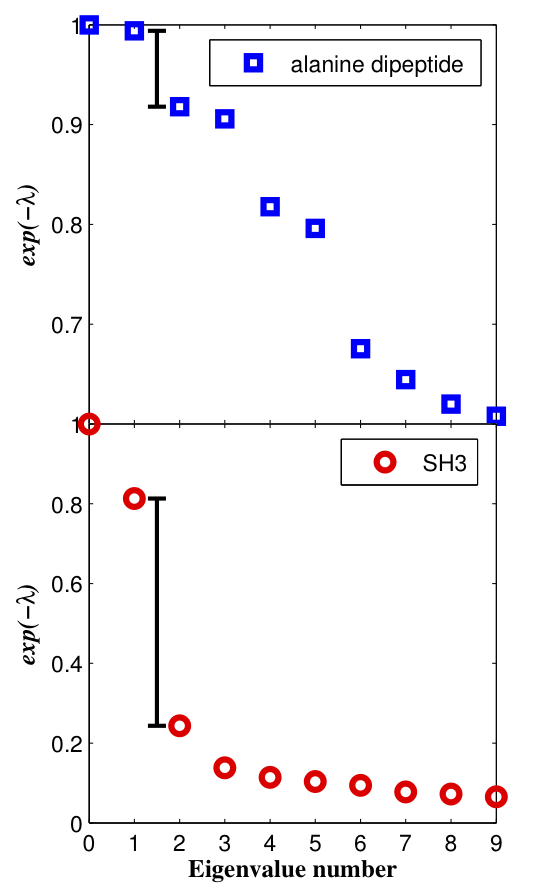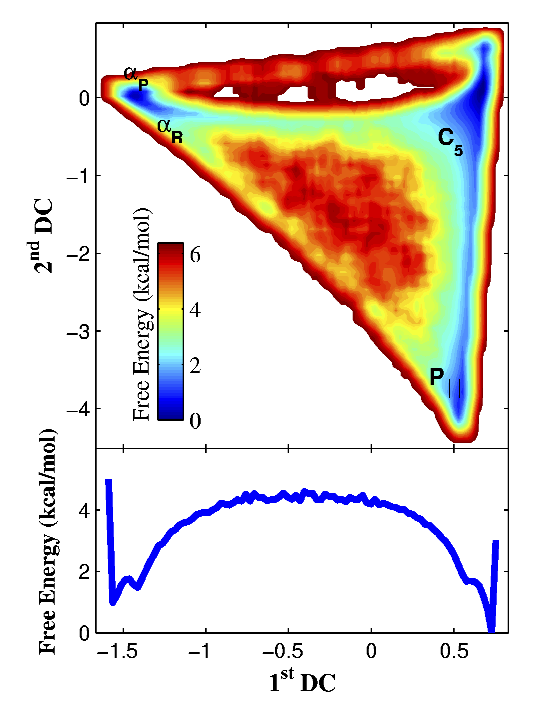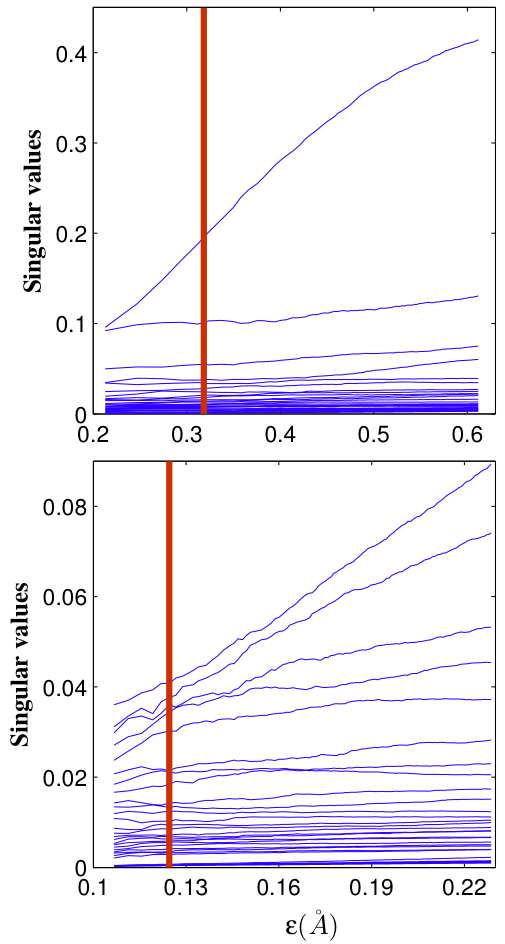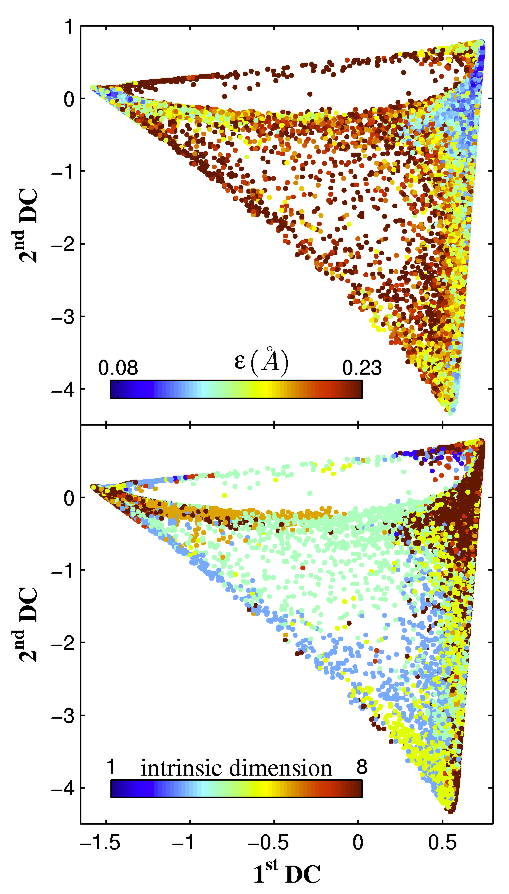## Links to pages of interest

CECAM workshops
Sridhar Mahadevan and myself have a few works on Markov Decision Processes. The tools of harmonic analysis on graphs, especially the eigenfunctions of the Laplacian and Diffusion Wavelets seem to find very natural applications to the study of Markov Decision Processes. First of all they can efficiently encode functions on general state spaces for these processes, thus performing a dimensionality reduction task which is recognized as fundamental in order to be able to perform efficient computation of these processes. Secondly there many connections between Markov Decision Processes and harmonic analysis of Markov Chains, stemming
from the connections between the Bellman equation and the Green’s function or fundamental matrix of a Markov Chain. Nonlinearity of the optimization problem and stochasticity of most ingredients in a Markov Decision Processes on the other hand offer new challanges. I will add more to this page as we make progress in this program.

### Papers

The most recent work on multiscale/hierarchical representations for MDP’s is this paper: Multiscale Markov Decision Problems: Compression, Solution, and Transfer Learning, J. Bouvrie, M. Maggioni.
A short version is available here: Efficient Solution of Markov Decision Problems with Multiscale Representations, J. Bouvrie and M. Maggioni, Proc. 50th Annual Allerton Conference on Communication, Control, and Computing, 2012.

In these works we introduce an automatic multiscale decomposition of MDP’s, which leads to a hierarchical set of MDP’s “at different scales” and corresponding to different portions of state space, separated by “geometric” and “task-specific” bottlenecks. The hierarchy of nested subproblems is such that each subproblem is itself a general type of MDP, that may be solved independently of the others (thereby giving trivial parallelization), and the solutions may then be stitched together through a certain type of “boundary-conditions”. These boundary conditions are propagated top to bottom, by solving the coarsest problem(s) first, and propagating down the solutions (essentially, these boundary conditions for finer-scale problems), and “filling-in” these coarse solutions by solving the finer problems with the propagated boundary conditions. Besides giving fast parallelizable algorithms for the solution of large MDP’s that are amenable to this decomposition, these decompositions also allow one to perform transfer learning of sub-problems (possibly at different scales), thereby enhancing the possibilities of transferability and power of transfer learning.

Here are electronic copies of two Technical Reports written with my collaborator Sridhar Mahadevan at the Computer Science Dept. at University of Mass. at Amherst about the application of the eigenfunctions of the Laplacian and Diffusion Wavelets to the solution of Markov Decision Processes:

Sridhar and myself organized a workshop on application of spectral methods to Markov Decision Processes, check out the page on our ICML
’06 Workshop
to be held during ICML 2006.

, with S. Mahadevan. Tech Report Univ. Mass. Amherst, CMPSCI 2006-35, May 2005.
This paper introduces a novel paradigm for solvingMarkov decision processes (MDPs), based on jointly learning representations and optimal policies. Proto-value functions are geometrically customized task-independent basis functions forming the building blocks of all value functions on a given state space graph or manifold. In this first of two papers, proto-value functions are constructed using the eigenfunctions of the (graph or manifold) Laplacian, which can be viewed as undertaking a Fourier analysis on the state space graph. The companion paper (Maggioni and Mahadevan, 2006) investigates building proto-value functions using a multiresolution manifold analysis framework called diffusion wavelets, which is an extension of classical wavelet representations to graphs and manifolds. Proto-value functions combine insights from spectral graph theory, harmonic analysis, and Riemannian manifolds. A novel variant of approximate policy iteration, called representation policy iteration, is described, which combines learning representations and approximately optimal policies. Two strategies for scaling proto-value functions to continuous or large discrete MDPs are described. For continuous domains, the Nystrom extension is used to interpolate Laplacian eigenfunctions to novel states. To handle large structured domains, a hierarchical framework is presented that compactly represents proto-value functions as tensor products of simpler proto-value functions on component subgraphs. A variety of experiments are reported, including perturbation analysis to evaluate parameter sensitivity, and detailed comparisons
of proto-value functions with traditional parametric function approximators.

, with S. Mahadevan. Tech Report Univ. Mass. Amherst, CMPSCI 2006-36. We present a novel hierarchical framework for solving Markov decision processes (MDPs) using a multiscale method called diffusion wavelets. Diffusion wavelet bases significantly differ from the Laplacian eigenfunctions studied in the companion paper (Mahadevan and Maggioni, 2006): the basis functions have compact support, and are inherently multi-scale both spectrally and spatially, and capture localized geometric features of the state space, and of functions on it, at different granularities in space- frequency. Classes of (value) functions that can be compactly represented in diffusion wavelets include piecewise smooth functions. Diffusion wavelets also provide a novel approach to approximate powers of transition matrices. Policy evaluation is usually the expensive step in policy iteration, requiring O(|S|^3) time to directly solve the Bellman equation (where |S| is the number of states for discrete state spaces or sample size in continuous spaces). Diffusion wavelets compactly represent powers of transition matrices, yielding a direct policy evaluation method requiring only O(|S|) complexity in many cases, which is remarkable because the Greenís function (I – P^\pi)-1 is usually a full matrix requiring quadratic space just to store each entry. A range of illustrative examples and experiments, from simple discrete MDPs to classic continuous benchmark tasks like inverted pendulum and mountain car, are used to evaluate the proposed framework.

Value Function Approximation with Diffusion Wavelets and Laplacian Eigenfunctions, with S. Mahadevan. Tech Report Univ. Mass. Amherst, CMPSCI 05-38, May 2005. Analysis of functions of manifolds and graphs is essential in many tasks, such as learning, classification, clustering, and reinforcement learning. The construction of efficient decompositions of functions has till now been quite problematic, and restricted to few choices, such as the eigenfunctions of the Laplacian on a manifold or graph, which has found interesting applications. In this paper we propose a novel paradigm for analysis on manifolds and graphs, based on the recently constructed diffusion wavelets. They allow a coherent and effective multiscale analysis of the space and of functions on the space, and are a promising new tool in classification and learning tasks, in reinforcement learning, among others. In this paper we overview the main motivations behind their introduction, their properties, and sketch a series of applications, among which multiscale document corpora analysis, structural nonlinear denoising of data sets and the tasks of value function approximation and policy evaluation in reinforcement learning, analyzed in two companion papers. The final form of this paper has appeared in the Proc. NIPS 2005with Sridhar Mahadevan, Tech Report Univ. Mass. Amherst, CMPSCI 05-39, May 2005.

Fast Direct Policy Evaluation using Multiscale Analysis of Markov Diffusion Processes, with Sridhar Mahadevan, accepted to ICML 2006.

Policy evaluation is a critical step in the approximate solution of large Markov decision processes (MDPs), typically requiring $O(|S|^3)$ to directly solve the Bellman system of $|S|$ linear equations (where $|S|$ is the state space size). In this paper we apply a recently introduced multiscale framework for analysis on graphs to design a faster algorithm for policy evaluation. For a fixed policy $\pi$, this framework efficiently constructs a multiscale decomposition of the random walk $P^\pi$ associated with the policy $\pi$. This enables efficiently computing medium and long term state distributions, approximation of value functions, and the {\it direct} computation of the potential operator $(I-\gamma P^\pi)^{-1}$ needed to solve Bellman’s equation. We show that even a preliminary non-optimized version of the solver competes with highly optimized iterative techniques, requiring in many cases a complexity of $O(|S|\log^2 |S|)$.

Learning Representation and Control in Continuous Markov Decision Processes, with Sridhar Mahadevan, Kimberly Ferguson, Sarah Osentoski, accepted to AAAI
2006. This paper presents a novel framework for simultaneously learning representation
and control in continuous Markov decision processes. Our approach builds on the framework of proto-value functions, in which the underlying representation or basis functions are automatically derived from a spectral analysis of the state space manifold. The proto-value functions correspond to the eigenfunctions of the graph Laplacian. We describe an approach to extend the eigenfunctions to novel states using the Nystr¨om extension. A least-squares policy iterationmethod is used to learn the control policy, where the underlying subspace for approximating the value function is spanned by the learned proto-value functions. A detailed set of experiments is presented using classic benchmark tasks, including the inverted pendulum and the mountain car, showing the sensitivity in performance to various parameters, and including comparisons with a parametric radial basis function method

Here are some links to useful pages:

2006 NSF Workshop and
Outreach Tutorials on Approximate Dynamic Programming
. Check out tutorials and workshop presentations.

### People

Links to some people in the field
(this is very very partial list and under continuous construction!):

These works are in collaboration with Rachael Brady and Eric Monson, in the Scientific Visualization group at Duke. Here is a list of posters and papers:
See Eric’s page on the FODAVA activites, which contains more materials and software.
Hyperspectral images are images with many colors: at each pixel location light is binned in many spectral bins (from tens to hundreds or more), thereby revealing chemical information about each pixel location. Throughout the years I have coauthored many papers investigating the use of hyperspectral imaging in a variety of applications, including medical diagnosis and anomaly detection in geoscience applications.

With many collaborators, we have written several papers during the years, focusing on supervised, semi-supervised and unsupervised algorithms for the analysis of hyperspectral imaging, ranging from automatic segmentation to anomaly detection.

Here I describe older work, dating to the beginning of the 2000’s, with Raphy Coifman and his research group, and Gus Davis (pathologist) on using DMD mirrors for tuning the light frequency patterns and designing classification algorithms for detection of carcinoma.  With light sources of increasingly broader ranges, spectral analysis of tissue sections has evolved from 2 wavelength image subtraction techniques to hyperspectral mapping. A variety of proprietary spectral splitting devices, including prisms and mirror, interferometer, variable interference filter-based monochromometer, tuned liquid crystals, mounted on microscopes in combination with CCD cameras and computers, have been used to discriminate among cell typeS, endogenous, exogenous pigments.

Goals We use a prototype unique tuned light source, a digital mirror array device (Plain Sight Systems) based on micro-optoelectromechanical systems, in combination with analytic algorithms
developed in the Yale Program in Applied Mathematics to evaluate the diagnostic efficiency of hyperspectral microscopic analysis of normal NAD neoplastic colon biopsies prepared as
microarray tissue sections. We compare the results to our previous spectral analysis of colon tissues and to other spectral studies of tissues and cells.

Experimental Details

Platform: The prototype tuned light digital mirror array device transilluminates H & E stained micro-array tissue
sections with any combination of light frequencies, range 440 nm – 700 nm, through a Nikon Biophot microscope. Hyper-spectral tissue images, multiplexed with a CCD camera (Sensovation), are captured & analyzed mathematically with a PC.

Image Source: 147 (76 normal & 71 malignant) hyperspectral gray
scale 400X images are derived from 68 normal and 62 malignant colon
biopsies selected from @ 200 normal and @600 malignant H & E
stained biopsies arrayed respectively on two different slides.

Cube:
Each hyperspectral image is a 3-D data cube (Figure 3) with spatial
coordinates x – 491 pixels, y – 653 pixels & spectral coordinates
z – 128 pixels, a total of 41 million transmitted spectra.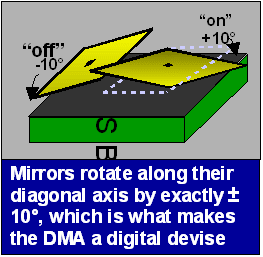Data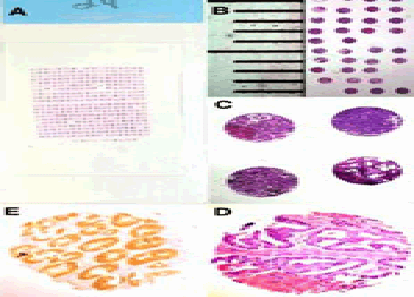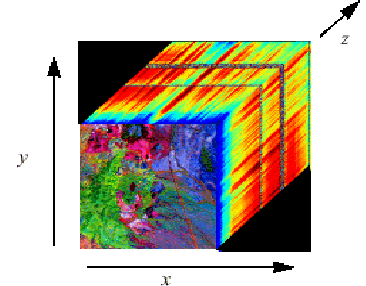Figure 2: Microarraybiopsies

Figure 3: Hyperspectral data cube (image from DataFusion Corp)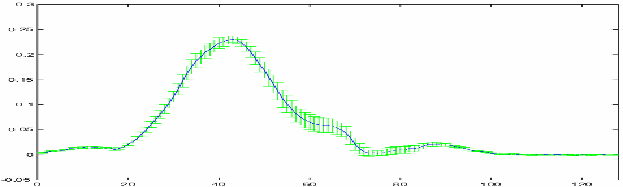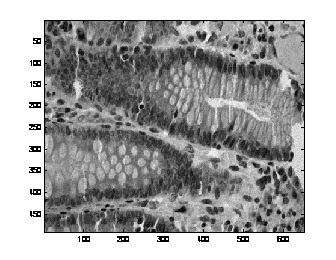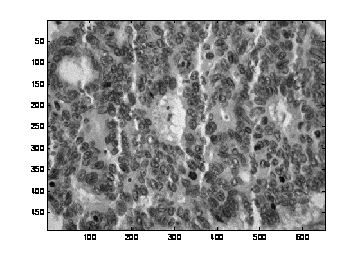Average nucleus spectrum with standard
deviation bars.

A spectral slice of a normal gland

A spectral slice of a cancerous gland

TISSUE CLASSIFICATION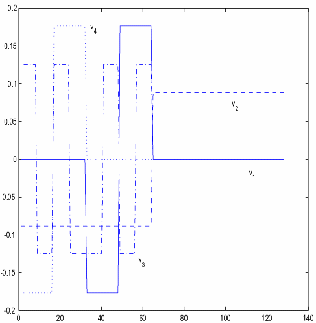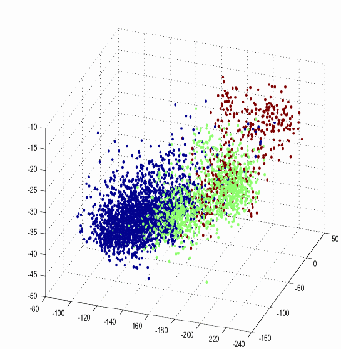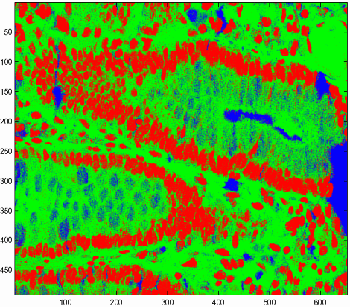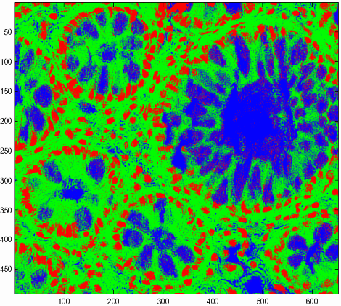Tissue classification algorithm on a sample

Tissue classification algorithm on a sample

ALGORITHM FOR
NORMAL/ABNORMAL DISCRIMINATION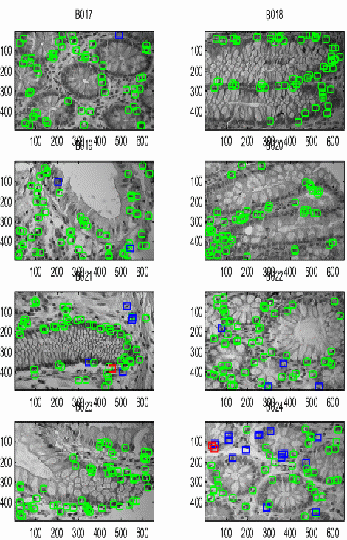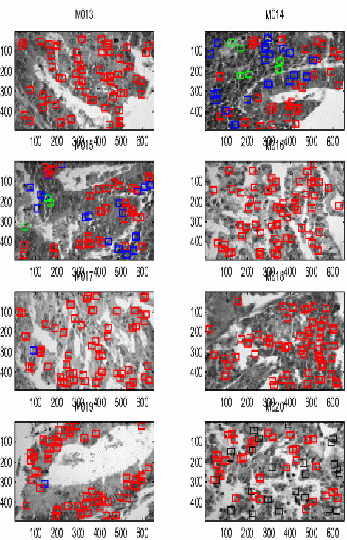Normal:

GREEN – true negative (normal classified as
normal);
BLUE – indeterminate
RED – false
positive (normal classified as abnormal)

Abnormal:

GREEN – false negative (abnormal classified as
normal)
BLUE – indeterminate
RED – true
positive (abnormal classified as abnormal)

Patches/Nuclei (8688) True Positive (4860) Table 1:Performance of classifier on nuclei patches, cross-validated. 94.0% (4568) 7.3% (280) 6.0% (292) 92.7% (3548)

CLASSIFICATION OF A
WHOLE SLIDE
The classification of a whole slide is obtained by selecting
40 random nuclei patches from the slide, and averaging the
corresponding classifications. The classification of the slides
has no mistakes, since the few errors of classification on the
nuclei are averaged out on the whole slide.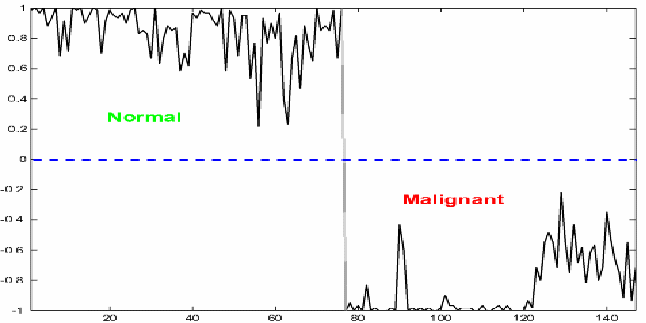A SPATIAL-SPECTRAL
CLASSIFIER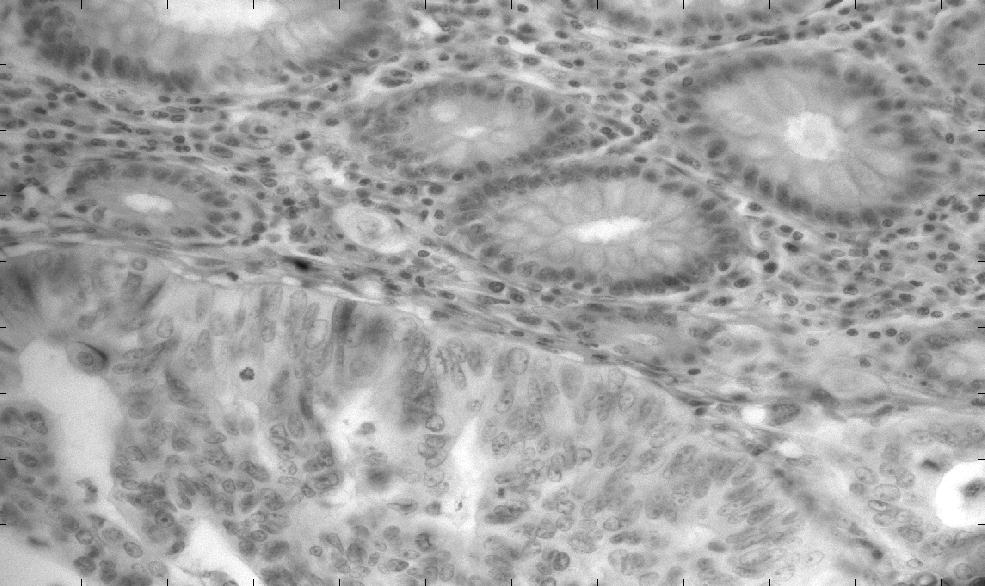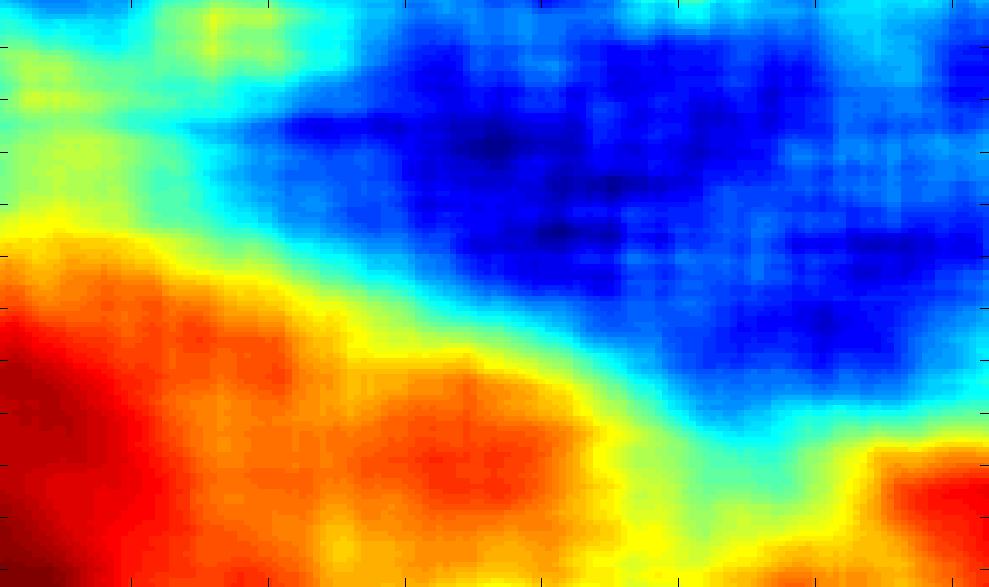## Papers

As an undergraduate students I studied wavelets under the guidance of Prof. Maurizio Soardi at the Universita’ degli Studi of Milano (now at the Bicocca site); I constructed some new biorthogonal wavelets, with dilation factors 2 and 3.

As a graduate student I studied harmonic analysis and wavelets under the guidance of Prof. Guido Weiss at Washington University in St. Louis; I constructed wavelet frames the discretize continuous wavelet transforms obtained from representations theory of groups and decompositions of unity on hypergroups.

Relevant publications

Designed and hosted by Prisma Analytics Inc.